References:

• T. Mitchell, 1997.
• R. Myers, R. Walpole, "Tests of Hypotheses", in R. Myers, R. Walpole, Probability and Statistics for Engineers and Scientists, Second Edition, Macmillan Publishing Co., Inc., New York, NY, 1978, pp. 268 - 273.
• P. Winston, 1992.

Rule Generation

Once a decision tree has been constructed, it is a simple matter to convert it into an equivalent set of rules.

Converting a decision tree to rules before pruning has three main advantages:

1. Converting to rules allows distinguishing among the different contexts in which a decision node is used.
• Since each distinct path through the decision tree node produces a distinct rule, the pruning decision regarding that attribute test can be made differently for each path.
• In contrast, if the tree itself were pruned, the only two choices would be:
1. Remove the decision node completely, or
2. Retain it in its original form.
2. Converting to rules removes the distinction between attribute tests that occur near the root of the tree and those that occur near the leaves.
• We thus avoid messy bookkeeping issues such as how to reorganize the tree if the root node is pruned while retaining part of the subtree below this test.
3. Converting to rules improves readability.
• Rules are often easier for people to understand.

To generate rules, trace each path in the decision tree, from root node to leaf node, recording the test outcomes as antecedents and the leaf-node classification as the consequent.

Rule Simplification Overview

Once a rule set has been devised:

1. Eliminate unecessary rule antecedents to simplify the rules.
• Construct contingency tables for each rule consisting of more than one antecedent.
• Rules with only one antecedent cannot be further simplified, so we only consider those with two or more.
• To simplify a rule, eliminate antecedents that have no effect on the conclusion reached by the rule.
• A conclusion's independence from an antecendent is verified using a test for independency, which is
• a chi-square test if the expected cell frequencies are greater than 10.
• Yates' Correction for Continuity when the expected frequencies are between 5 and 10.
• Fisher's Exact Test for expected frequencies less than 5.
2. Eliminate unecessary rules to simplify the rule set.
• Once individual rules have been simplified by eliminating redundant antecedents, simplify the entire set by eliminating unecessary rules.
• Attempt to replace those rules that share the most common consequent by a default rule that is triggered when no other rule is triggered.
• In the event of a tie, use some heuristic tie breaker to choose a default rule.

Contingency Tables

The following is a contingency table, a tabular representation of a rule.

 C1 C2 Marginal Sums R1 x11 x12 R1T = x11 + x12 R2 x21 x22 R2T = x21 + x22 Marginal Sums CT1 = x11 + x21 CT2 = x12 + x22 T = x11 + x12 + x21 + x22

R1 and R2 represent the Boolean states of an antecedent for the conclusions C1 and C2
(C2 is the negation of C1).
x11, x12, x21 and x22 represent the frequencies of each antecedent-consequent pair.
R1T, R2T, CT1, CT2 are the marginal sums of the rows and columns, respectively.

The marginal sums and T, the total frequency of the table, are used to calculate expected cell values in step 3 of the test for independence.

Test for Independence

Given a contingency table of dimensions r by c (rows x columns):

1. Calculate and fix the sizes of the marginal sums.

2. Calculate the total frequency, T, using the marginal sums.

3. Calculate the expected frequencies for each cell.

The general formula for obtaining the expected frequency of any cell xij, 1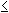ir, 1jc in a contingency table is given by: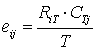where RiT and CTj are the row total for ith row and the column total for jth column.

4. Select the test to be used to calculate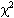based on the highest expected frequency, m:

 if then use m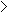10 Chi-Square Test 5m10 Yates' Correction for Continuity m5 Fisher's Exact Test

5. Calculateusing the chosen test.

6. Calculate the degrees of freedom.

df = (r - 1)(c - 1)

7. Use a chi-square table withand df to determine if the conclusions are independent from the antecedent at the selected level of significance,.
• Assume= 0.05 unless otherwise stated.

• If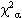• Reject the null hypothesis of independence and accept the alternate hypothesis of dependence.
• We keep the antecedents because the conclusions are dependent upon them.
• If• Accept the null hypothesis of independence.
• We discard the antecedents because the conclusions are independent from them.

Chi-Square Formulae